## 问题定义

1. 触发词检测：触发词（Trigger）是事件抽取中的重要信息，一般是出现在事件提及中，最能明确表达发生事件的词，一般是动词或名词。例如，"20年前的春天，他出生了"一句中，"出生"为该事件提及文本中的事件触发词。
2. 事件类型检测：即通过分类等方式得到事件的类型，由于触发词在事件中的关键性，因此也可以被视作触发词类型检测。事件的类型取决于事件模式的设计，或事理图谱schema的设计。例如，某事件模式中将"咬伤"、"砍死"等触发词的事件定义为"伤害"类型的事件。
3. 事件论元抽取：事件论元（Event Argument）指的是事件中的参与者，包含实体、时间、数值、文本等数据组成。例如，"张三在2022年成功晋升"中的张三、2022年均为"晋升"事件的事件论元。
4. 论元角色识别：根据事件模式或事理图谱的定义，将抽取的事件论元按照其在事件中扮演的具体角色进行分类。例如，在某"公安事理图谱"中的"张三"、"李四"等人在其对应的犯罪记录中均为"加害者"这一事件角色。

1. 事件分割：也有称故事分割，给定一段文本（如新闻、论坛发言等），检测出不同事件的边界。例如，在央视"今日新闻简讯"中包含了当日多条要闻，有的新闻条目使用了多段文本描述，有的文本段落中一次包含了多条新闻，将它们分离成独立的事件文本片段即为本任务的目标。
2. 事件发现：在新闻、论坛发言等文本中，检测出新的事件（New Event Detection）。常用于舆情系统等应用。
3. 事件追踪：在新闻、论坛发言等文本中，检测同属于之前的已有事件的文本片段，通过此方式追踪事件（Event Tracking）的发展情况。常用于舆情系统等应用。

## 基准数据集

ACE05 是事件抽取任务最常用的基准数据集，包含了英语、阿拉伯语和汉语的精标注信息抽取数据，囊括了事件抽取中几个最常见的子任务。ACE05 遵循 LDC（Linguistic Data Consortium）的用户协议，需要注册为LDC会员才能下载与使用。

## 常用方法与典型技术

### 基于模式匹配的事件抽取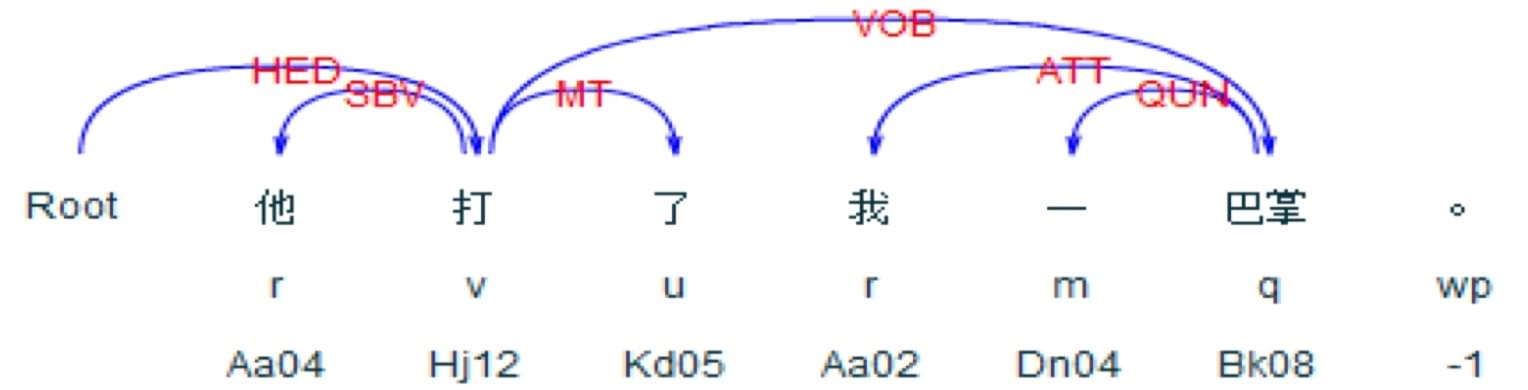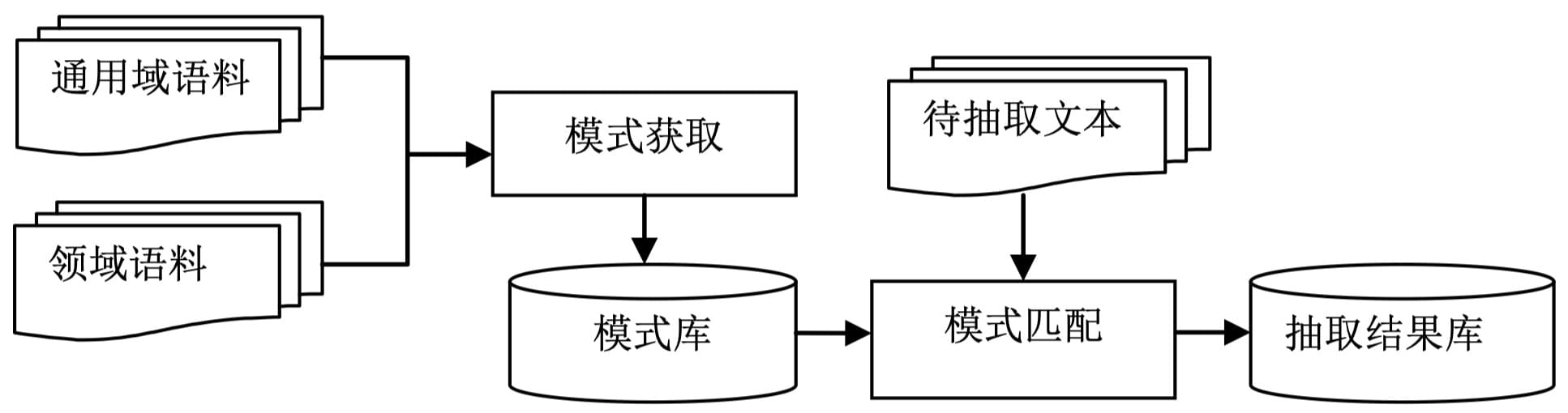### 基于机器学习技术的事件抽取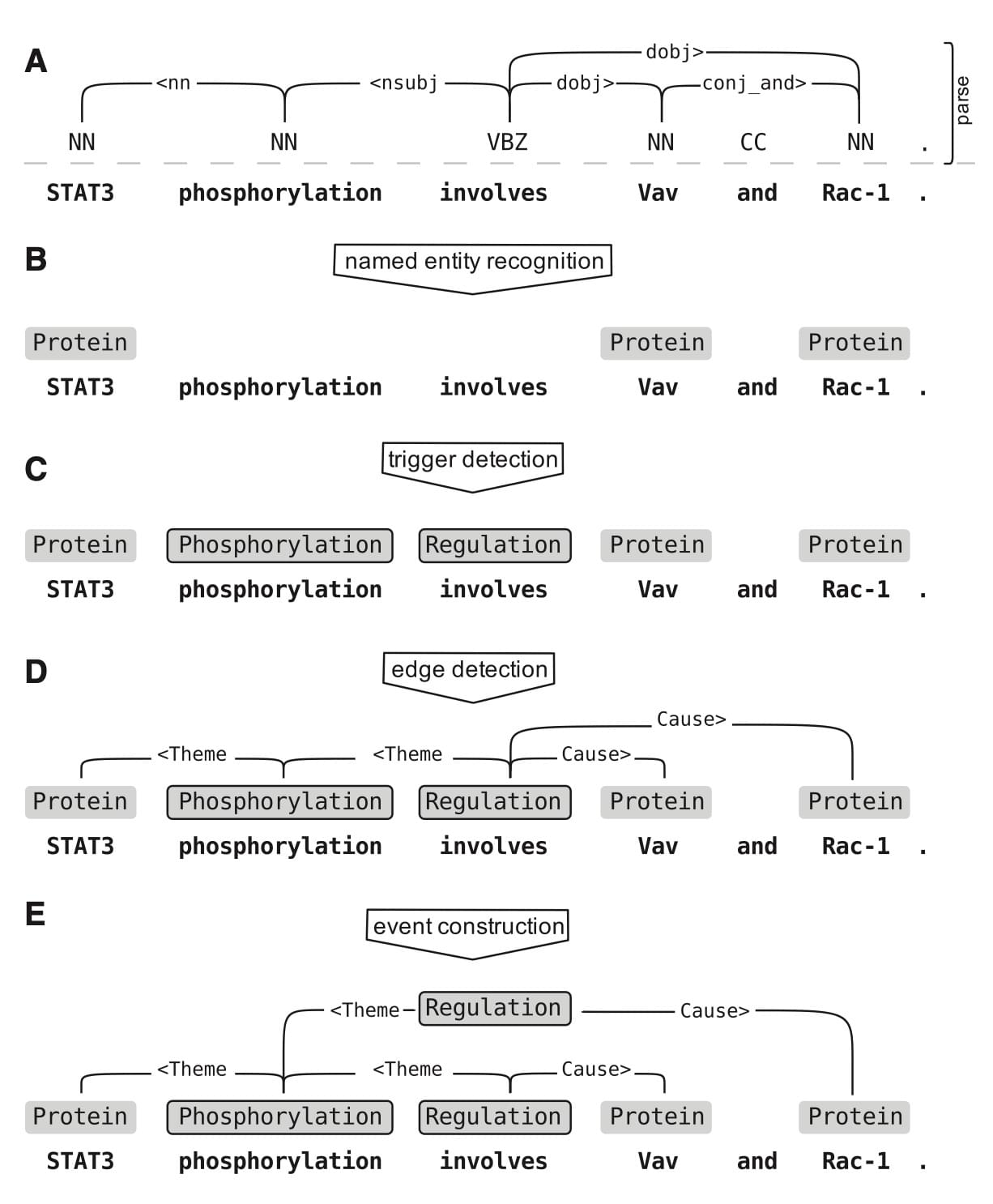### 基于深度学习技术的事件抽取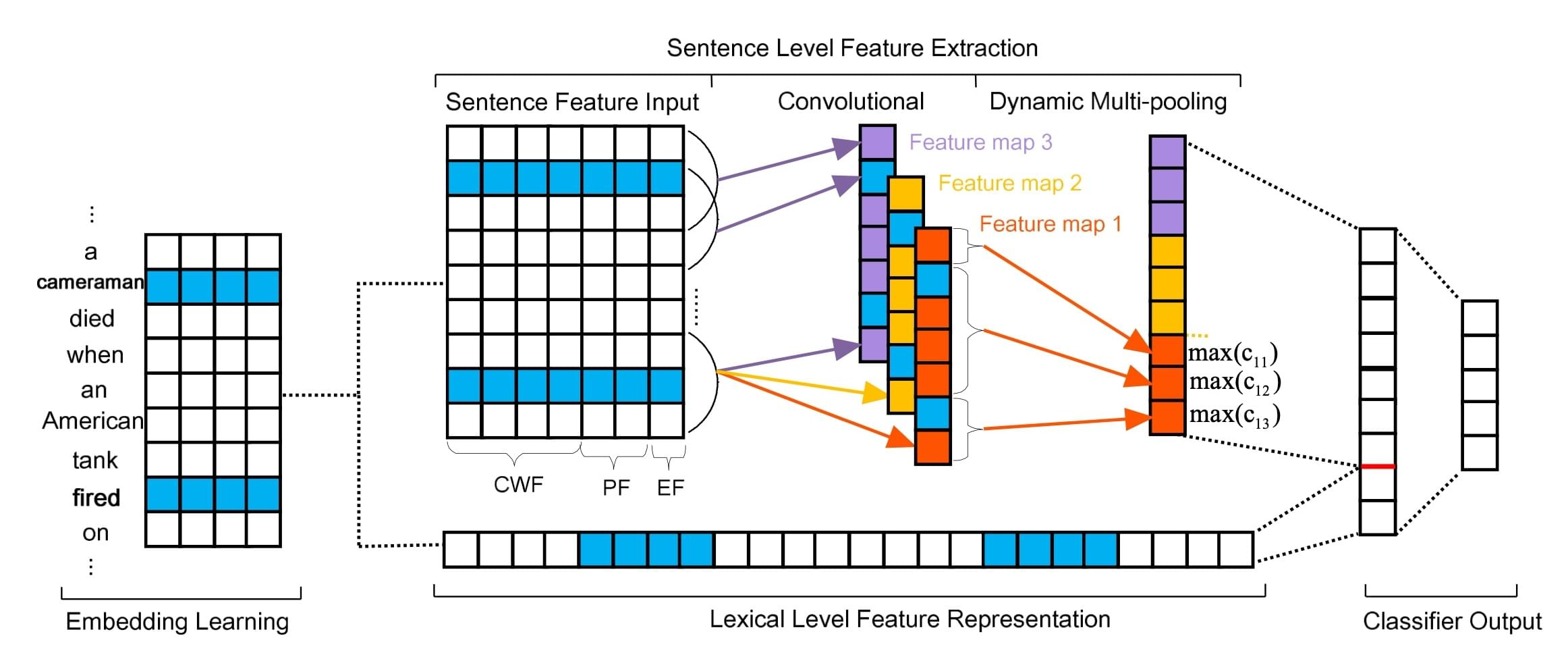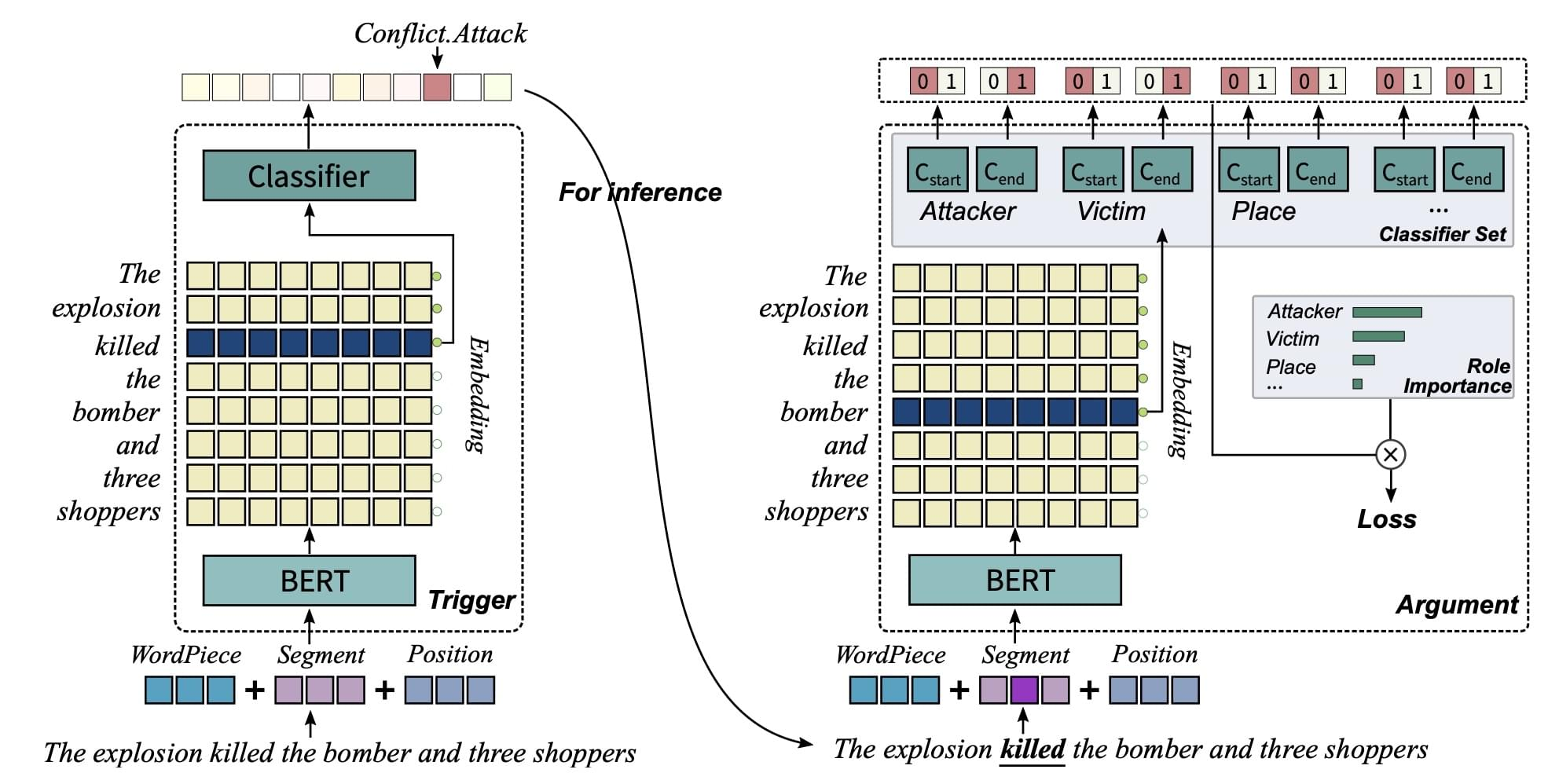## 事件抽取技术发展趋势

### 小样本事件抽取范式

$L=L_{\text {query }}+\beta \hat{L}_{\text {intra }}+\gamma \hat{L}_{\text {inter }}$

$L_{i n t r a}=\sum_{i=1}^{N} \sum_{k=1}^{K} \sum_{j=k+1}^{K} \operatorname{mse}\left(v_{i}^{j}, v_{i}^{k}\right)$

L_inter 为类间损失，让不同聚类距离（即类心原型距离）越远越好：

$L_{i n t e r}=1-\sum_{i=1}^{N} \sum_{j=i+1}^{N} \operatorname{cosine}\left(c_{i}, c_{j}\right)$

L_query 为query 损失，让 query 能尽量与正确对应的原型距离更近：

$\begin{gathered} L_{q u e r y}(x, S)=-\log P(y=t \mid x, S) \\ P\left(y=t_{i} \mid x, S\right)=\frac{\exp \left(-d\left(f(q, p), \mathbf{c}^{i}\right)\right)}{\sum_{j=1}^{N} \exp \left(-d\left(f(q, p), \mathbf{c}^{j}\right)\right)} \end{gathered}$

### 基于问答的事件抽取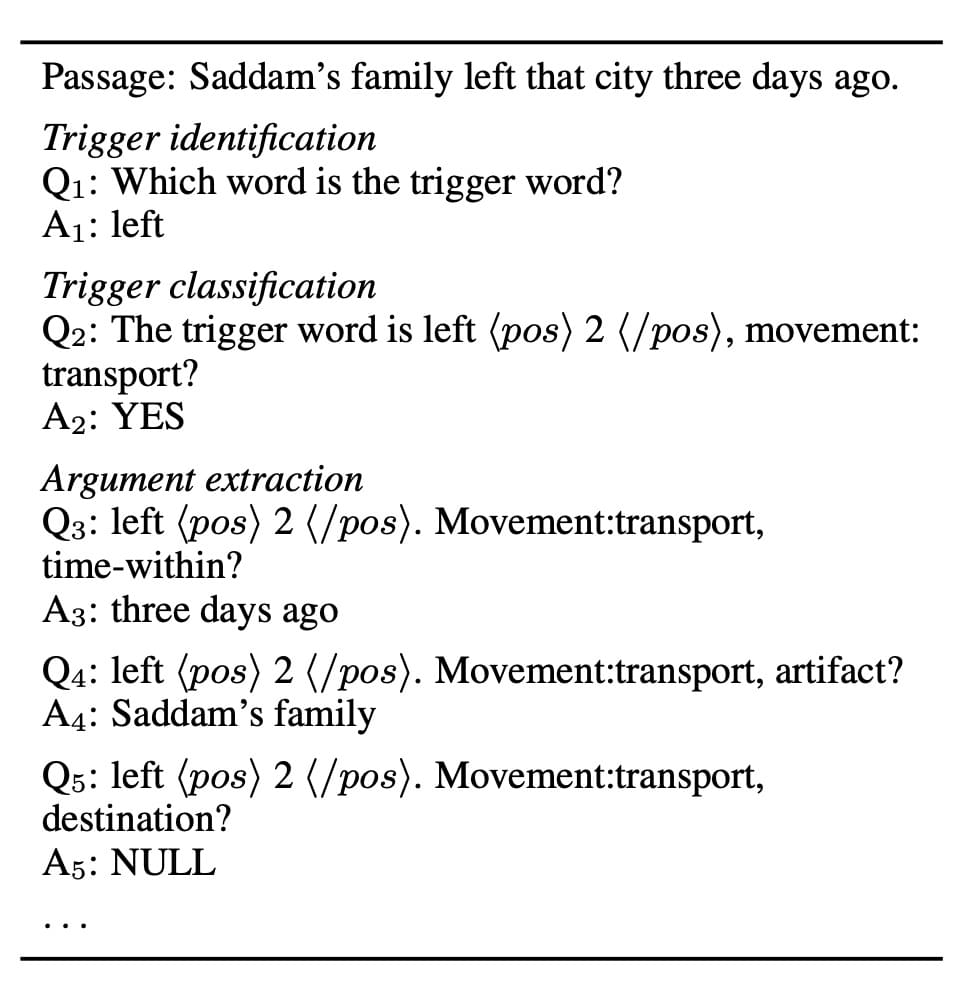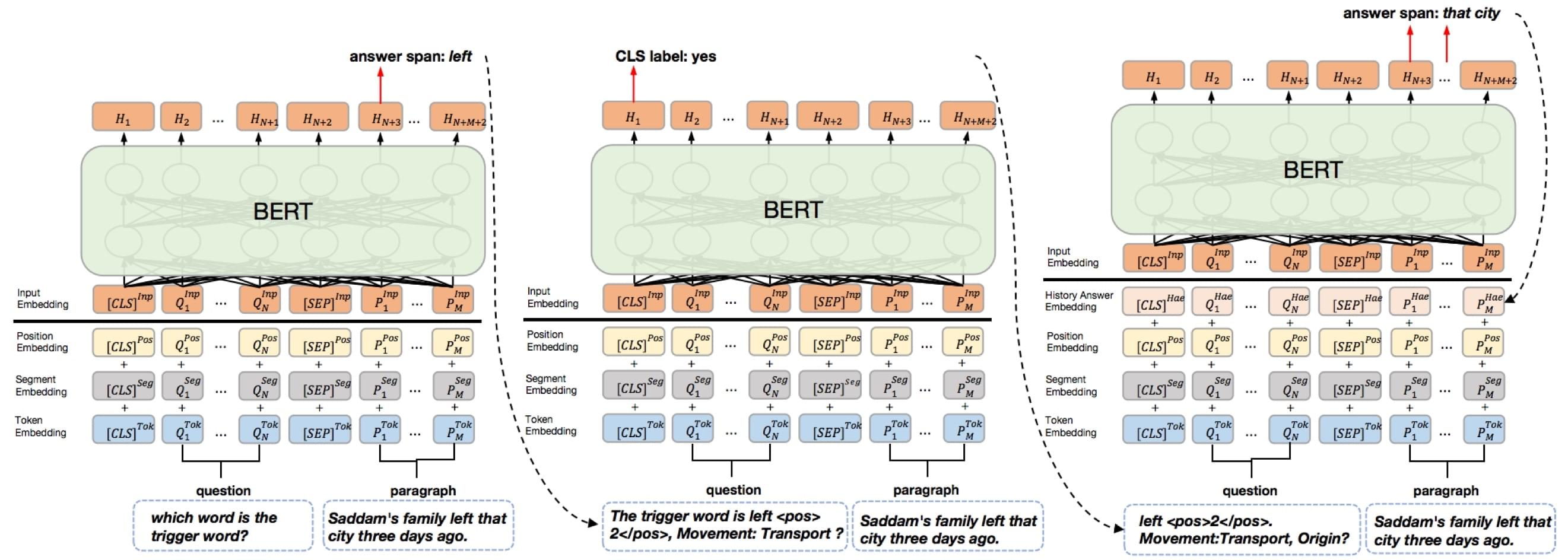### 面向特定数据的事件抽取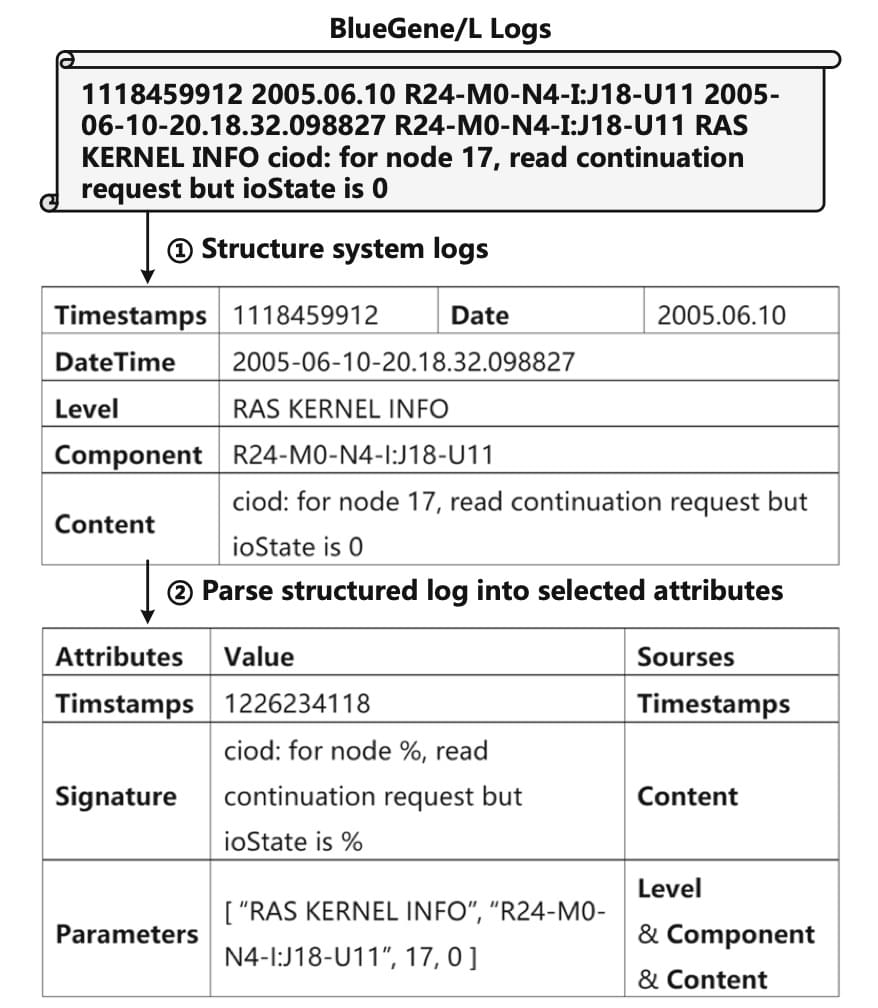## Reference

•  ACE2005 Dataset:  http://projects.ldc.upenn.edu/ace/
•  Chen et al, Event extraction via dynamic multi-pooling convolutional neural networks, ACL 2015
• 项威 王邦, 中文事件抽取综述, 计算机技术与发展 2020, 卷2
•  Björne et al, Complex event extraction at PubMed scale, Bioinformatics (2010), 26
•  Yang et al, Exploring pre-trained language models for event extraction and generation, ACL 2019
•  Li et al, Event Extraction as Multi-turn Question Answering, EMNLP 2020
•  Sheng et al, Casee: A joint learning framework with cascade decoding for overlapping event extraction, ACL 2021
•  Liu et al, An overview of event extraction and its applications, arxiv 2021